RS Aggarwal Test: Real Numbers - 3

# RS Aggarwal Test: Real Numbers - 3 - Class 10

Test Description

## 25 Questions MCQ Test Mathematics (Maths) Class 10 - RS Aggarwal Test: Real Numbers - 3

RS Aggarwal Test: Real Numbers - 3 for Class 10 2023 is part of Mathematics (Maths) Class 10 preparation. The RS Aggarwal Test: Real Numbers - 3 questions and answers have been prepared according to the Class 10 exam syllabus.The RS Aggarwal Test: Real Numbers - 3 MCQs are made for Class 10 2023 Exam. Find important definitions, questions, notes, meanings, examples, exercises, MCQs and online tests for RS Aggarwal Test: Real Numbers - 3 below.
Solutions of RS Aggarwal Test: Real Numbers - 3 questions in English are available as part of our Mathematics (Maths) Class 10 for Class 10 & RS Aggarwal Test: Real Numbers - 3 solutions in Hindi for Mathematics (Maths) Class 10 course. Download more important topics, notes, lectures and mock test series for Class 10 Exam by signing up for free. Attempt RS Aggarwal Test: Real Numbers - 3 | 25 questions in 25 minutes | Mock test for Class 10 preparation | Free important questions MCQ to study Mathematics (Maths) Class 10 for Class 10 Exam | Download free PDF with solutions
 1 Crore+ students have signed up on EduRev. Have you?
RS Aggarwal Test: Real Numbers - 3 - Question 1

### Pairs of natural numbers whose least common multiple is 78 and the greatest common divisor is 13 are:

Detailed Solution for RS Aggarwal Test: Real Numbers - 3 - Question 1

Let the no.s be a & b,

GCD [ a , b ] = 13;
=> Let a = 13m and b = 13n

Now, LCM [ a , b ] = 78
=> 13m | 78
=> m | 6 and similarly, n | 6; --> Also, m does not divide n

.'. Possible values of (m,n) are :->
[ 1 , 6 ] , [ 2 , 3 ]
.'. Possible values for ( a , b ) are : [ 13 , 78 ] , [ 26 , 39 ]

RS Aggarwal Test: Real Numbers - 3 - Question 2

### 1/√3 is -

Detailed Solution for RS Aggarwal Test: Real Numbers - 3 - Question 2

Let as assume to the contrary that 1/✓3 is rational number. Then, we can find coprime integers a and b (≠ 0) such that :
1/✓3= P/Q { where P and Q are co-prime and Q not equal to 0}
✓3 P =Q .1
✓3 = Q/P
Since Q/P is a Rational number

Since L.H.S = R.H.S

So ✓3 is also rational number.

But this contradicts the fact that ✓3 is an irrational number. This contradiction has arisen because of our incorrect assumption that 1/√3 is a rational number.

So this proves that 1/√3 is an irrational number.

RS Aggarwal Test: Real Numbers - 3 - Question 3

### 7√3 is -

Detailed Solution for RS Aggarwal Test: Real Numbers - 3 - Question 3

√3 cannot be expressed in the form p/q where q ≠ 0 which is the form of a rational number. So any number multiplied by it will give an irrational number. So 7√3 is irrational.

RS Aggarwal Test: Real Numbers - 3 - Question 4

5√3 is -

Detailed Solution for RS Aggarwal Test: Real Numbers - 3 - Question 4

To prove : 5-√3 is irrational

Proof:
Let us assume that 5 -√3 is rational.

Let ,
5 - √3 = r , where "r" is rational

5 - r = √3
Here,
LHS is purely rational.But,on the other hand ,RHS is irrational.
Hence,5-√3 is irrational.

RS Aggarwal Test: Real Numbers - 3 - Question 5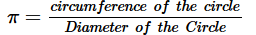Detailed Solution for RS Aggarwal Test: Real Numbers - 3 - Question 5

In the 1760s, Johann Heinrich Lambert proved that the number π (pi) is irrational: that is, it cannot be expressed as a fraction a/b, where a is an integer and b is a non-zero integer.

RS Aggarwal Test: Real Numbers - 3 - Question 6

If two positive integers p and q are written as p=a2b2 and q=a3b, a,b are prime numbers then verify that L.C.M.(p,q)×H.C.F.(p,q)=pq

Detailed Solution for RS Aggarwal Test: Real Numbers - 3 - Question 6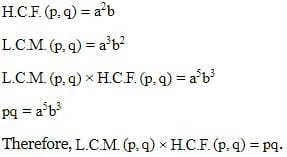RS Aggarwal Test: Real Numbers - 3 - Question 7

HCF (p,q,r) · LCM (p,q,r) =

Detailed Solution for RS Aggarwal Test: Real Numbers - 3 - Question 7

Since HCF(p,q,r)*LCM(p,q,r) is not equal to pq/r, neither it is equal to qr/p and neither is p,q,r. So the correct answer is D . Also, HCF(p,q,r)*LCM(p,q,r) is not equal to p*q*r . This condition only holds for two numbers.

RS Aggarwal Test: Real Numbers - 3 - Question 8

Two natural numbers whose sum is 85 and the least common multiple is 102 are:

Detailed Solution for RS Aggarwal Test: Real Numbers - 3 - Question 8

Prime factorisation of 102 = 2 x 3 x 17.
Prime factorisation of 85 = 5 x 17 = (2+3) x 17.
The two numbers are:
1. 2 x 17 = 34.
2. 3 x 17 =51

RS Aggarwal Test: Real Numbers - 3 - Question 9

0.737373...=

Detailed Solution for RS Aggarwal Test: Real Numbers - 3 - Question 9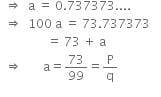⇒ a = 0.737373...
⇒  100a = 73.737373
= 73 + a
⇒ a = 73/99 = p/q
⇒  p=73 and q = 99 are co-prime.
Here, q=3X 11.

RS Aggarwal Test: Real Numbers - 3 - Question 10

If p is a positive prime integer, then √p is –

Detailed Solution for RS Aggarwal Test: Real Numbers - 3 - Question 10

Let us assume, to the contrary, that √p is
rational.
So, we can find coprime integers a and b(b ≠ 0)
such that √p = a/b
⇒ √p b = a
⇒ pb2 = a2 ….(i) [Squaring both the sides]
⇒ a2 is divisible by p
⇒  a is divisible by p
So, we can write a = pc for some integer c.
Therefore, a2 = p2c2 ….[Squaring both the sides]
⇒ pb2 = p2c2 ….[From (i)]
⇒ b2 = pc2
⇒ b2 is divisible by p
⇒ b is divisible by p
⇒ p divides both a and b.
⇒ a and b have at least p as a common factor.
But this contradicts the fact that a and b are coprime.
This contradiction arises because we have
assumed that √p is rational.
Therefore, √p is irrational.

RS Aggarwal Test: Real Numbers - 3 - Question 11

LCM of three numbers 28, 44, 132 is –

Detailed Solution for RS Aggarwal Test: Real Numbers - 3 - Question 11

LCM = 22. 71. 111. 31 = 924

RS Aggarwal Test: Real Numbers - 3 - Question 12

If a is a positive integer and p be a prime number and p divides a2, then

Detailed Solution for RS Aggarwal Test: Real Numbers - 3 - Question 12

If a is a positive integer and p be a prime number and p divides a2
So it implies that p divides a .

RS Aggarwal Test: Real Numbers - 3 - Question 13

If the HCF of 65 and 117 is expressible in the form 65m – 117, then the value of m is

Detailed Solution for RS Aggarwal Test: Real Numbers - 3 - Question 13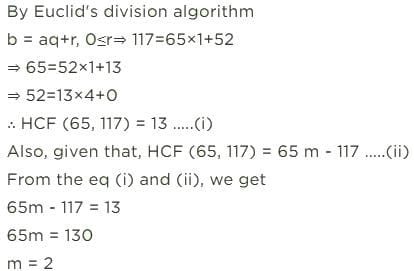RS Aggarwal Test: Real Numbers - 3 - Question 14

If x =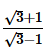and y =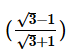, then the value of (x2 + y2) is

Detailed Solution for RS Aggarwal Test: Real Numbers - 3 - Question 14

The correct option is Option A.

x = √3 + 1 / √3 - 1

y = √3 - 1 / √3 + 1

Now,

x² + y²

= (√3 + 1 / √3 - 1)² + (√3 - 1 / √3 + 1)²

= (3 + 2√3 + 1 / 3 - 2√3 + 1) + (3  - 2√3 + 1 / 3 + 2√3 + 1)

= (4 + 2√3 / 4 - 2√3) + (4 - 2√3 / 4 + 2√3)

= (4 + 2√3)² + (4 - 2√3)² / (4 - 2√3) × (4 + 2√3)

By solving this,

= 28 + 16√3 + 28 - 16√3 / 16 - 12

= 56 / 4

= 14

RS Aggarwal Test: Real Numbers - 3 - Question 15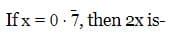Detailed Solution for RS Aggarwal Test: Real Numbers - 3 - Question 15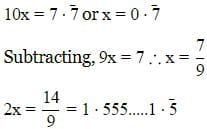RS Aggarwal Test: Real Numbers - 3 - Question 16

Find the value of x then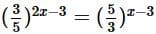Detailed Solution for RS Aggarwal Test: Real Numbers - 3 - Question 16

(3/5)2x-3= (5/3)x-3
(3/5)2x-3=(3/5)3-x
2x-3=3-x
2x+x=6
3x=6
x=2

RS Aggarwal Test: Real Numbers - 3 - Question 17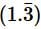is equal to –

RS Aggarwal Test: Real Numbers - 3 - Question 18

The product of 4√6 and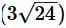is -

Detailed Solution for RS Aggarwal Test: Real Numbers - 3 - Question 18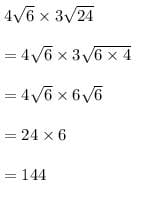RS Aggarwal Test: Real Numbers - 3 - Question 19

If two positive integers a and b are written as a = x3y2 and b = xy3; x, y are prime numbers, then HCF (a, b) is

Detailed Solution for RS Aggarwal Test: Real Numbers - 3 - Question 19

Given that, a =x3y2 = x × x × x × y × y
and b = xy3 = x × y × y × y
∴ HCF of a and b = HCF (x3y2,xy3) = x × y × y = xy
[Since, HCF is the product of the smallest power of each common prime factor involved in the numbers]

RS Aggarwal Test: Real Numbers - 3 - Question 20

The least number that is divisible by all the numbers from 1 to 10 (both inclusive) is

Detailed Solution for RS Aggarwal Test: Real Numbers - 3 - Question 20

The least number divisible by all the numbers from 1 to 10 will be the LCM of these numbers.

We have,

1 = 1

2 = 2 × 1

3 = 3 × 1

4 = 2 × 2

5 = 5 × 1

6 = 2 × 3

7 = 7 × 1

8 = 2 × 2 × 2

9 = 3 × 3

10 = 2 × 5

So, LCM of these numbers = 1 × 2 × 2 × 2 × 3 × 3 × 5 × 7 = 2520

Hence, least number divisible by all the numbers from 1 to 10 is 2520.

RS Aggarwal Test: Real Numbers - 3 - Question 21

What will be the least possible number of the planks, if three pieces of timber 42 m, 49 m and 63 m long have to be divided into planks of the same length?

Detailed Solution for RS Aggarwal Test: Real Numbers - 3 - Question 21

The lengths of three pieces of timber are 42m, 49m and 63m respectively.

We have to divide the timber into equal length of planks.

∴ Greatest possible length of each plank = HCF (42, 49, 63)

Prime factorization:

42 = 2 × 3 × 7

49 = 7 × 7

63 = 3 × 3 × 7

∴HCF = Product of the smallest power of each common prime factor involved in the numbers = 7

Hence, the greatest possible length of each plank is 7m.

RS Aggarwal Test: Real Numbers - 3 - Question 22

The value of i37+ 1/i67 is –

Detailed Solution for RS Aggarwal Test: Real Numbers - 3 - Question 22

i37+1/i67
=(i2)18.i + 1/(i2)33.i
=1.i + 1/-1.i         (Since i2=-1)
=i -1/i
=(i- 1)/i
=(-1-1)/i
=-2/i
Now, multiplying by i/i,
-2/i . i\i
=-2i/i2
=-2i/-1
=2i

RS Aggarwal Test: Real Numbers - 3 - Question 23

The standard form of (1 + i) (1 + 2i) is –

Detailed Solution for RS Aggarwal Test: Real Numbers - 3 - Question 23

( 1 + i ) (1 + 2i )

=1 + 2i + i + 2i^2

=1 + 3i -2  ( Because i^2 = -1)

= -1 + 3i

= a + ib = -1 + 3i

Here a = -1 and b = 3

So the standard form of  (1 + i) (1 + 2i) is :

-1 + 3i

So option D is correct answer.

RS Aggarwal Test: Real Numbers - 3 - Question 24

The conjugate of 4 – 5i is

Detailed Solution for RS Aggarwal Test: Real Numbers - 3 - Question 24

The conjugate of the complex number a+bi is a−bi.
In order to find the complex conjugate, you just reverse the sign of the imaginary part of the number ; so it is 4+5i

RS Aggarwal Test: Real Numbers - 3 - Question 25

The multiplicative inverse of 1 – i is

Detailed Solution for RS Aggarwal Test: Real Numbers - 3 - Question 25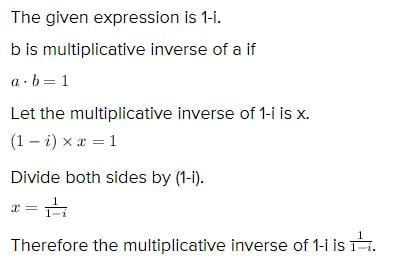## Mathematics (Maths) Class 10

115 videos|478 docs|129 tests
Information about RS Aggarwal Test: Real Numbers - 3 Page
In this test you can find the Exam questions for RS Aggarwal Test: Real Numbers - 3 solved & explained in the simplest way possible. Besides giving Questions and answers for RS Aggarwal Test: Real Numbers - 3, EduRev gives you an ample number of Online tests for practice

## Mathematics (Maths) Class 10

115 videos|478 docs|129 tests